# .css-4zleql{display:block;}std::steam# 100 Days Of ML Code — Day 052

Jehoshaphat I. Abu
·Aug 30, 2018·

## Recap From Day 051

Day 051, we looked at working with time; how dynamic time warping works. We learned that Dynamic time warping was designed specifically to compute the distance between two sequences of data. Just like we’ve seen, each data point can consist of several features. Perhaps X and Y values from a mouse, or pitch, or roll, and yaw values from a Wiimote, or even MFCCs from audio.

Today, we will continue from where we left off in day 051

## Working with time

### How Dynamic Time Warping Works continued

…is what is used by our nearest neighbor algorithm to compute the distance between examples in feature space if we have some number of features N.

The image below shows how we could use Euclidean distance to compute the similarity, or rather, dissimilarity, between two sequences.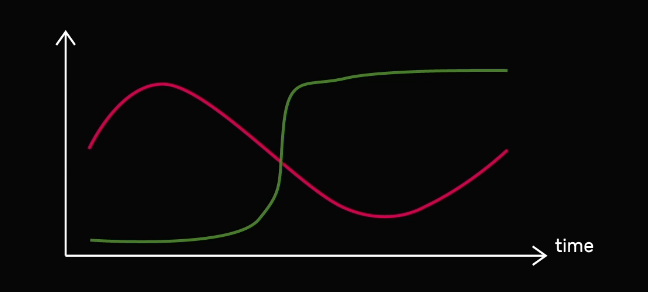Source

If our two sequences are the same length, we could compute the Euclidean distance from the first point in one sequence to the first point in another sequence, and we could do the same for the second point in each sequence and the third and so on.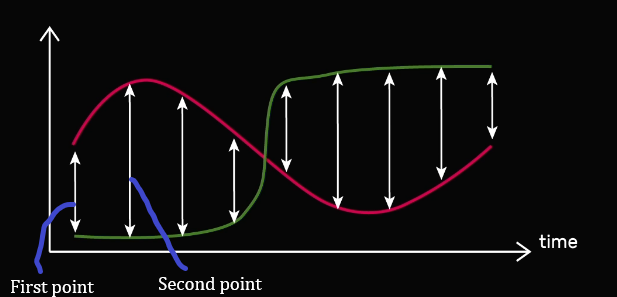Source

The image below contains two sequences, A and B. On the X axis, we have time, and on the Y axis, we have a single feature value. We can think of this as a measurement of a hand height over time as the hand move in front of a kinect, for instance.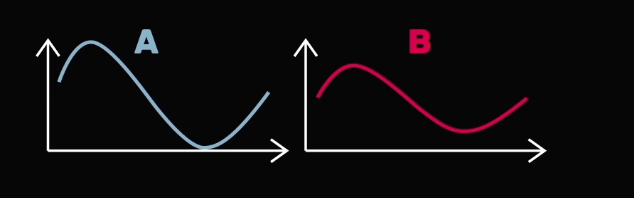Source

In both of these sequences, the hand is moved up then down then back up. So how similar are they using Euclidean distance? Let’s start by computing the distance from point one of line A to point one of line B, then the distance from point two of line A to point two of line B, all the way to the final point in each line as shown below.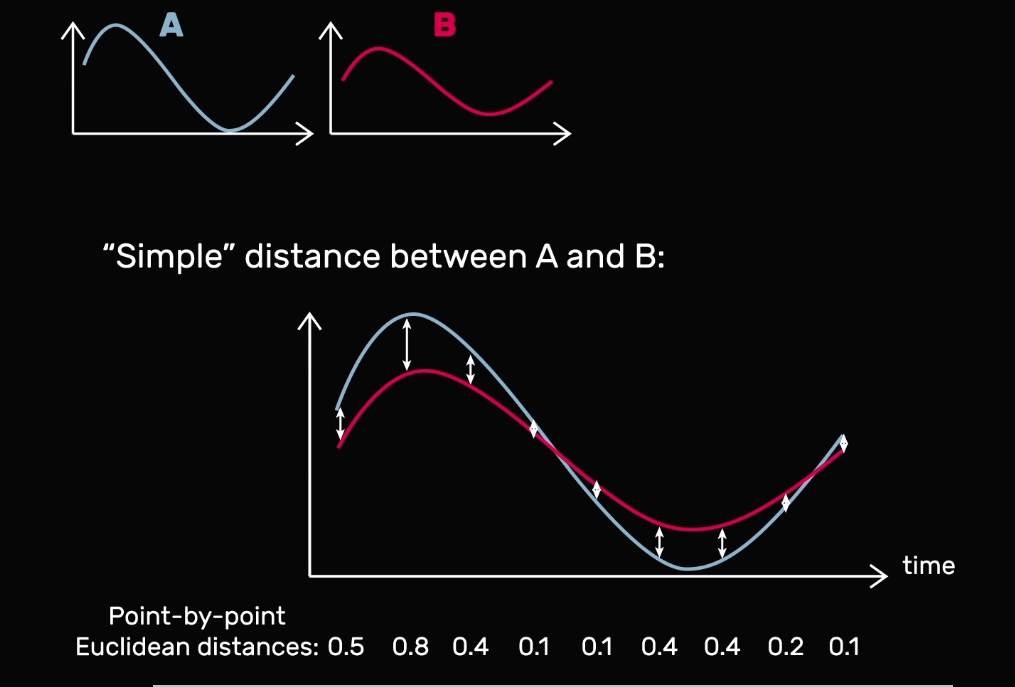Source

Each of these calculations is measuring how close the two sequence are at a single moment in time. In order to get a single distance measurement indicating the overall distance between the two sequences, we can just add these up as shown below.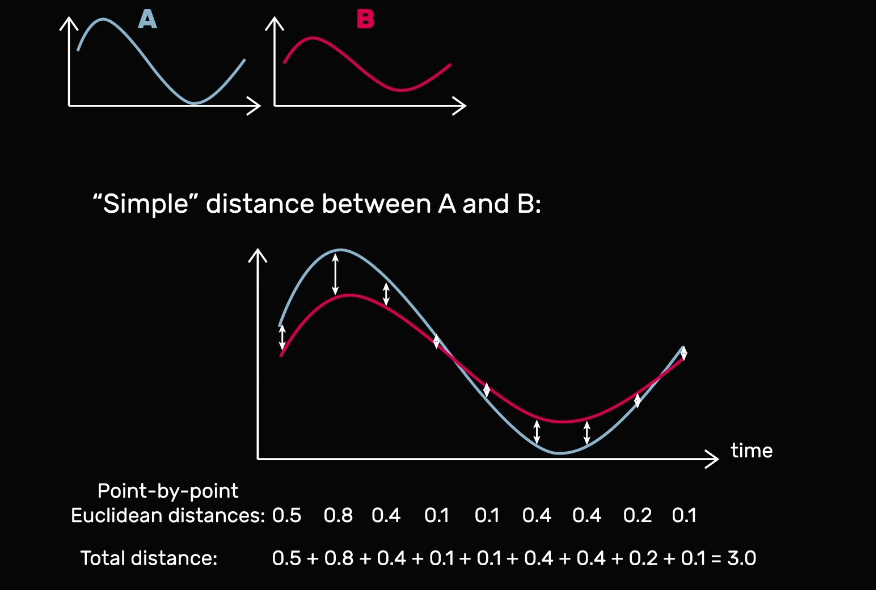Source

After all, we can reasonably assume that if two sequence are very close at all moments in time, like sequences A and B in the image above, we would say that they are very similar overall, so the distance between them should be low.

On the other hand, if we repeat this process to compare A to another sequence, C, we see that A and C have a larger overall distance as shown below. It seems sensible.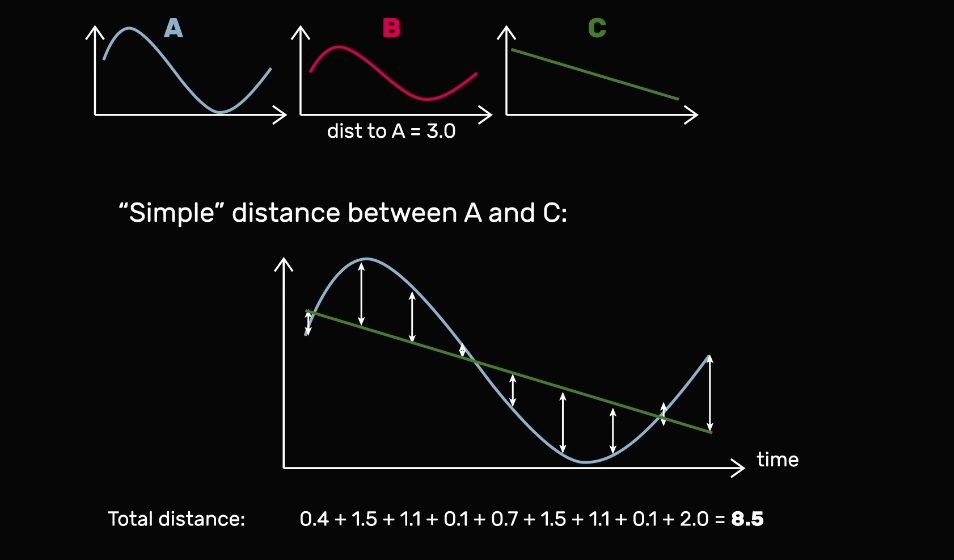Source

The hand wave in C would look quite different from the hand wave in A and B. However, what if we take sequence B and shift it over just a bit to make a new sequence, D? That question will be answered tomorrow.

That’s all for day 052. I hope you found this informative. Thank you for taking time out of your schedule and allowing me to be your guide on this journey. And until next time, be legendary.

Reference